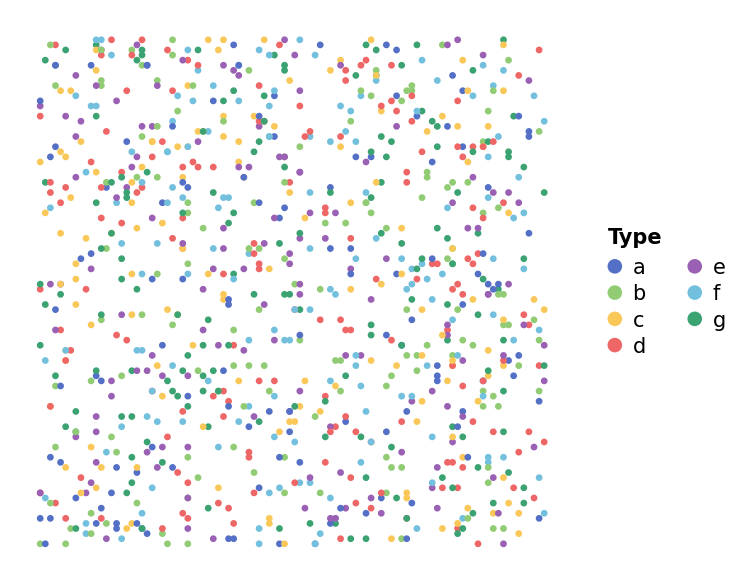# Point map Example (Categorical data)#

Here shows how to draw the point map

```import numpy as np
import milkviz as mv
```

## First let’s create some random data#

```np.random.seed(0)
xy = np.random.randint(0, 100, (1000, 2))
types = np.random.choice(list("abcdefg"), 1000)
```

## Create the point map#

```mv.point_map(xy, types=types,
legend_kw={"title": "Type", "ncol": 2})
``````<AxesSubplot: >
```